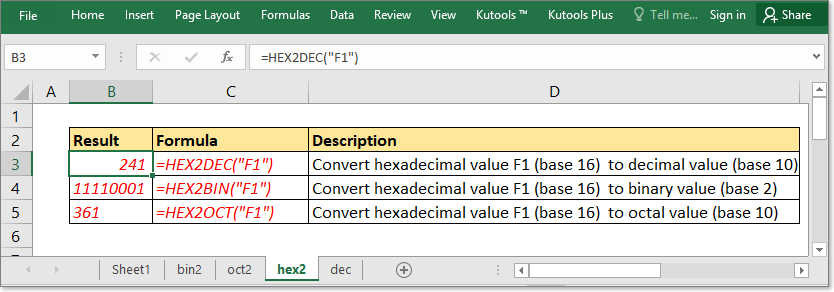Tip: Other languages are Google-Translated. You can visit the English version of this link.

## Convert hexadecimal value to decimal or binary or octal value### Convert hexadecimal value to decimal value

Generic formula 1:

 =HEX2DEC(text)

Arguments

 Text: Required, the text representation of number you want to convert it from hexadecimal (base 16) to decimal (base 10) value.

Examples

 Formula Description Result =HEX2DEC("F1") Convert hexadecimal value F1 to decimal value 241 =HEX2DEC(B3) (Cell B3 contains 1F) Convert hexadecimal value 1F to decimal value 31

Generic formula 2:

 =DECIMAL(text,16)

Arguments

 Text: Required, the text representation of number you want to convert it from octal (base 16) to decimal (base 10) value, and the text needed to be enclosed with quotation marks. 16: Required, the octal base.

Examples

=DECIMAL(“F1”,8)

Explanation:

Convert hexadecimal value F1 to decimal value.

Return

Press Enter key to return 241.

### Convert hexadecimal value to binary value

Generic formula:

 =HEX2BIN(text)

Arguments

 Text: Required, the text representation of number you want to convert it from hexadecimal (base 16) to binary (base 2) value,and the text needed to be enclosed with quotation marks.

Examples

=HEX2BIN(“F1”)

Explanation:

Convert hexadecimal value F1 to binary value.

Return

Press Enter key to return 11110001.

### Convert hexadecimal value to octal value

Generic formula:

 =HEX2OCT(text)

Arguments

 Text: Required, the text representation of number you want to convert it from hexadecimal (base 16) to octal (base 8) value, and the text needed to be enclosed with quotation marks.

Examples

=HEX2OCT(“F1”)

Explanation:

Convert hexadecimal value F1 to octal value.

Return

Press Enter key to return 361.

### Remarks

The Text argument in formulas must be in hexadecimal value formatting, which means the character elements must be in the scale of 0-9 and A-F, or it returns #NUM! error value.

#### Relative Functions

• Excel DECIMAL function
The DECIMAL function converts a text representation of a number in a base into its equivalent decimal number. Take a instance, 11 is a text representation of 3 in base 2, using the formula =DECIMAL(11,2) convert 11 into decimal number 3.

### Excel Productivity Tools

Would you like to complete your daily work quickly and perfectly? Kutools for Excel brings 300 powerful advanced features (Combine workbooks, sum by color, split cell contents, convert date, and so on...) and save 70% time.

• Designed for 1500 work scenarios, helps you solve 80% Excel problems.
• Reduce thousands of keyboard and mouse clicks every day, relieve your tired eyes and hands.
• Become an Excel expert in 3 minutes. No longer need to remember any painful formulas and VBA codes.
• 60-day unlimited free trial. 60-day money back guarantee. Free upgrade and support for 2 years.

#### Brings Efficient Tabs to Office (include Excel), Just Like Chrome, Firefox, And New IE

• Office Tab Increases your productivity by 50% when viewing and editing multiple documents.
• Reduce hundreds of mouse clicks for you every day, say goodbye to mouse hand.
• One second to switch between dozens of open documents!Say something here...
symbols left.